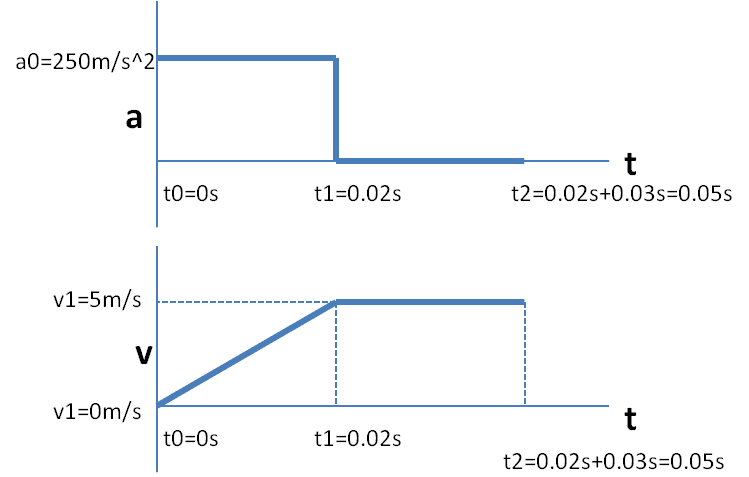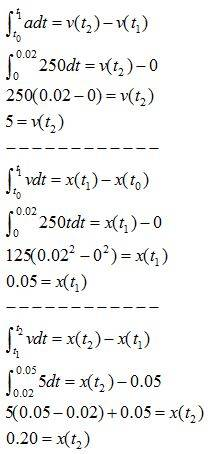# New to physics and need help.

I'm sure some of you will have a good laugh about this problem but its driving me bonkers! here goes: chameleons catch insects by rapidly extending their tongues. A chameleon's tongue accelerates at 250(m/s^2) for 20 ms (milliseconds i presume) then travels at constant speed for another 30 ms. during the total time 1/20 seconds how far does the tongue reach?
this was all the information given for the problem.

I have used kinematic equations before in my class but the professor moves from subject to subject without segue. During some point I'm sure he addressed problems like this but i just don't know what to do or how to start. any takers?

## Answers and Replies

Hi,

While I'm waiting for my question to be answered, I thought I'd help you out. Is the answer 0.25 meters? I might be completely off, but like I said, just trying to help haha.

Try to think about the situation

Givens

Vo = 0 m/s ( Tongue at rest initially)
a = 250 m/s^2
t1 = 20 ms or 20*10^-3 s = 0.02 s

Find the distance utilizing the kinematic equation

x1 = Vot + 1/2 at^2
x1 = 1/2 at^2
x1 = 1/2(250)(0.02)^2

Now it goes after this at 30 ms or 0.03 s

Now we know the that the final velocity of the trip till it accelerates is equal the constant velocity it travels during the second part of the non - accelerated motion.

V = Vo+at
V = at
V = 250*0.02
V constant during the 0.03 s is 250*0.02 m/s
The time during this period is 0.03 s

Since a= 0

x2 = vt
x2 = 250*0.02*0.03

x1+x2 = 1/2(250)(0.02)^2 + 250*0.02*0.03
Δx = 1/2(250)(0.02)^2 + 250(0.02)(0.03) ( m)

Since i think your not supposed to give out the answers i will leave it that.

Variables used.

Vo = Initial. Maybe you might use u or Vi or V1.
V = Final/constant velocity . You might also use V or Vf or V2 in the normal conventions
x = displacement. You might also use d or x or s depending on the conventions

Hope that helps you. it is ok if you struggle with physics at first. But visualizing the problem really helps rather than plugging the right equation! Hope i have helped. Good luck.

Edit: It comes to 0.2m for me:) So your slightly off i think, but not way off.

Last edited:
Assuming linear motion, v0 (initial velocity) = 0m/s, a=250m/s^2, t=20ms
find Vfinal (after the 20ms) using v=v0 +at, V= 0m/s + (250m/s^2)(20ms)
then use that Vfinal from after the 20ms to calculate the distance of the 30ms part,
delta X = [(250m/s^2)(20ms)](30ms) which gives you the distance that the tongue traveled during the 30 ms.
So just calculate the distance the tongue traveled in the first 20ms using:
delta X = Vi * t + 1/2*a*t^2
delta X = 0*t + 1/2*(250m/s^2)(20ms)^2

so your answer should be:
1/2*(250m/s^2)(20ms)^2 + [(250m/s^2)(20ms)](30ms)

I just learned this stuff 2 days ago myself, but I hope this is correct, and I hope it helps.

Yeah it's 0.20m. Made an calculation error :P.

Because I am such an awesome guy (and still waiting for my question to be answered), I decided to graphically show how I did this problem without using the kinematic equations (I hate those equations).Incredible gadget the internet is; thanks all for the advice esp. Astro. Obviously I wanted the method not the answer. yes, this is linear motion in one dimension.

Dr. strangelove you may want to check your numbers/units again all that figures to be 1.25x10^5m which is impractically large considering the body length of a Jackson's chameleon is only about 40 cm including the tail.

Dr. strangelove you may want to check your numbers/units again all that figures to be 1.25x10^5m which is impractically large considering the body length of a Jackson's chameleon is only about 40 cm including the tail.
never mind i misread ms as m/s for whatever reason.

No problem bucky! It is a 2 step kinematic problem.

@Airforce: Amazing graphical approach. Very intuitive. How did you manage to create these graphs. Is it generated from a website or is there a software available. I tend to use graphical approach for much more intensive problems. This is a fairly simple 2 step kinematics problem:) So i use the kinematics equation blindly. Plus i haven't learned calculus based physics yet.

Dr strange love hasn't been consistent with his units. He forgot to convert ms to s.

Dr. strangelove you may want to check your numbers/units again all that figures to be 1.25x10^5m which is impractically large considering the body length of a Jackson's chameleon is only about 40 cm including the tail.

Because you actually looked up the length of a Jackson's chameleon, I created a lizard just for you to applaud your enthusiastic attitude:No problem bucky! It is a 2 step kinematic problem.

@Airforce: Amazing graphical approach. Very intuitive. How did you manage to create these graphs. Is it generated from a website or is there a software available. I tend to use graphical approach for much more intensive problems. This is a fairly simple 2 step kinematics problem:) So i use the kinematics equation blindly. Plus i haven't learned calculus based physics yet.

Dr strange love hasn't been consistent with his units. He forgot to convert ms to s.

For the graphs, I used Powerpoint. It took a long time though. Next time I'll probably use Solidworks or Autocad, which is a complete overkill but it'll get the job done much, much quicker. For the text, I used MathType. I agree it is a simple kinematics problem, but I was just offering another approachYou will learn calculus based physics in college if you're an engineering or physics major like I am.

wow! I am going to grade 12 this year (2 days). I am also very interested in majoring in physics. The graphs looks so professional . Never guessed it could have been powerpoint haha!

use something like:

x1=1/2at^2 when t1=2E-2seconds

x2=vt2 where t2=3E-2 and v is constant given

x3=vt3 where t3=1/20seconds minus 5E-2seconds and v is constant given

and Xtotal= x1+x2+x3 which should be your answer

make sense?

haha, I actually owned a jacksons chameleon for about 6 years so I knew that figure off-hand, nice lizard clip art though.